# Average Tricks and Practice Questions - Quantitative Aptitude

An average, or more accurately an arithmetic mean is, in crude terms, the sum of n different data divided by n.
For example, if a batsman scores 35, 45 and 37 runs in first, second and third innings respectively, then his average runs in 3 innings is equal to (35+45+37)/3 = 39 runs.

Therefore, the two mostly used formulas are:#### Ques 1.

The average age of 30 boys of a class is equal to 14 yrs. When the age of the class teacher is included the average becomes 15 yrs. Find the age of the class teacher.
Solution: Total ages of 30 boys = 14 × 30 = 420 yrs.
Total ages when class teacher is included = 15 × 31 = 465 yrs.
Age of class teacher = 465 - 420 = 45 yrs.

#### Direct formula:

Age of new entrant = New average + No. of old members × increase in average
= 15 + 30 (15 – 14) = 45 yrs.

#### Ques 2.

The average weight of 4 men is increased by 3 kg when one of them who weighs 120 kg is replaced by another man. What is the weight of the new man?
Solution: Quicker approach: If the average is increased by 3 kg, then the sum of weighs increases by 3 × 4 = 12 kg.

And this increase in weight is due to the extra weight included due to the inclusion of new person.
Weight of new person = 120 + 12 = 132 kg.

Direct formula:
Weight of new person = weight of removed person + No. of persons × increase in average = 120 + 12 × 3 = 132 kg.

#### Ques 3.

The average of marks obtained by 120 candidates in a certain examination is 35. If the average marks of passed candidates is 39 and that of the failed candidates is 15, what is the number of candidates who passed the examination?#### Ques 4.

The average of 11 results is 50. If the average of first six results is 49 and that of last six is 52, find the sixth result.
Solution: The total of 11 results = 11 × 50 = 550
The total of first 6 results = 6 × 49 = 294
The total of last 6 results = 6 × 52 = 312
The 6th result is common to both;

Therefore, Sixth result = 294 + 312 – 550 = 56#### Ques 5.

The average age of 8 persons in a committee is increased by 2 years when two men aged 35 yrs and 45 yrs are substituted by two women. Find the average age of these two women.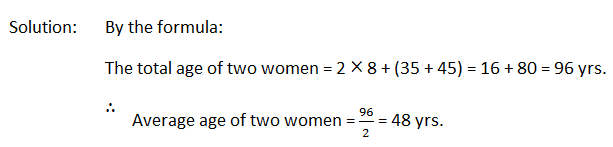#### Ques 6.

The average age of a family of 6 members is 22 years. If the age of the youngest member be 7 yrs, then what was the average of the family at the birth of the youngest member?#### Ques 7.

A man bought 13 shirts of Rs 50 each, 15 pants of Rs 60 each and 12 pairs of shoes at Rs 65 a pair. Find the average value of each article.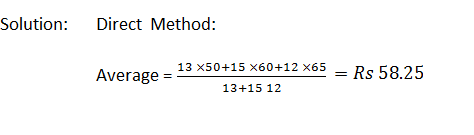#### Ques 8.

The average score of a cricketer in two matches is 27 and in three other matches is 32. Then find the average score in all the five matches.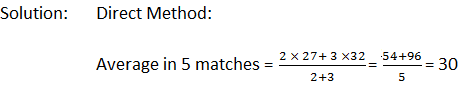#### Ques 9.

The average of 11 results is 30, that of the first five is 25 and that of the last five 28. Find the value of the 6th number.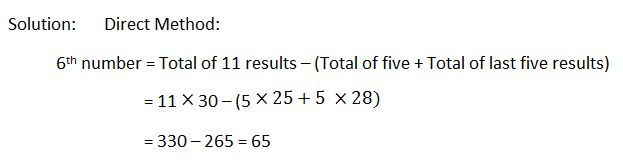#### Ques 10.

In a class, there are 20 boys whose average age is decreased by 2 months, when one boy aged 18 yrs is replaced by a new boy. Find the age of the new boy.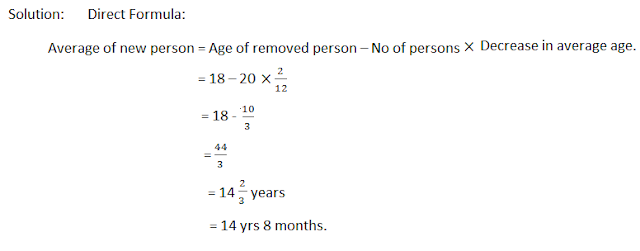#### Ques 11.

A batsman in his 17th innings makes a score of 85, and thereby increases his average by 3. What is his average after 17 innings?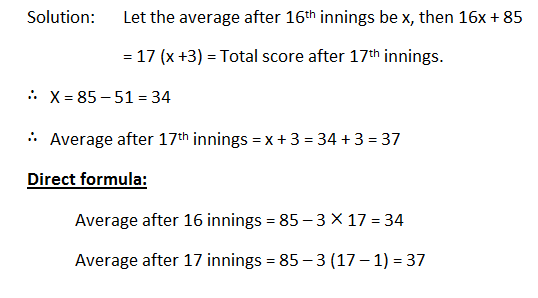#### Ques 12.

A cricketer has completed 10 innings and his average is 21.5 runs. How many runs must he make in his next innings so as to raise his average to 24?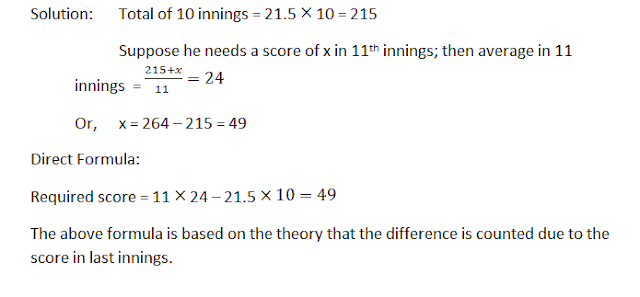#### Latest Courses on BankExamsToday1.